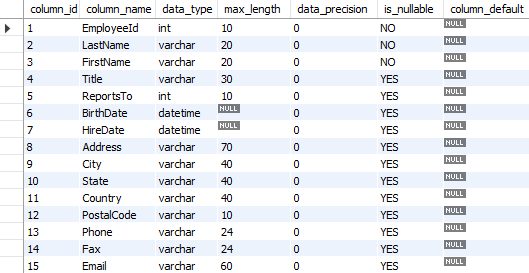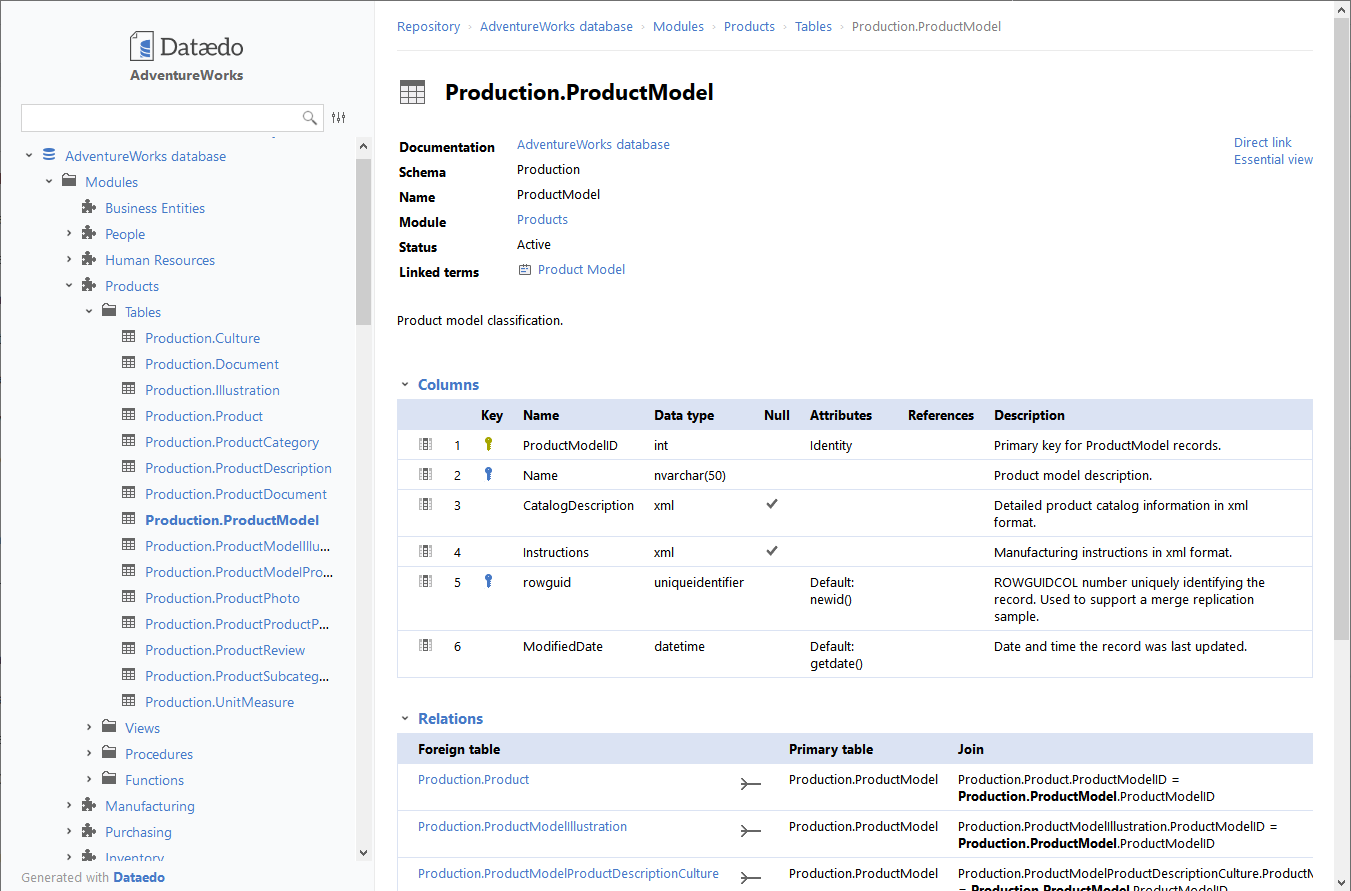## List all columns in specific table in MariaDB database

Article for:

Query below returns a list of all columns in a specific table in MariaDB.

## Query

``````select ordinal_position as column_id,
column_name as column_name,
data_type as data_type,
case when numeric_precision is not null
then numeric_precision
else character_maximum_length end as max_length,
case when datetime_precision is not null
then datetime_precision
when numeric_scale is not null
then numeric_scale
else 0 end as data_precision,
is_nullable,
column_default
from information_schema.columns
where table_name = 'table name' -- put table name here
--    and table_schema = 'schema name' -- put schema name here
order by ordinal_position;
``````

## Columns

• column_id - column position in table, starting at 1
• column_name - name of a column in a table
• data_type - column data type
• max_length - data type max length
• data_precision - data type precision
• is_nullable - if column is nullable then YES, else NO
• column_default - Default expression of the column

## Rows

• One row represents a single column
• Scope of rows: represent all columns in a specific table
• Ordered by column's ordinal position

## Sample results## You could also get this

Get this interactive HTML data dictionary in minutes with Dataedo.See live HTML data dictionary sample